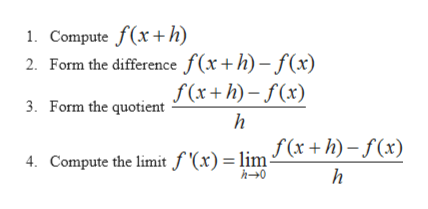# use the four step process to find f'(x) and then find f'(1), f'(2), and f'(3) f(X)= 2-3X2

Question
13 views

use the four step process to find f'(x) and then find f'(1), f'(2), and f'(3)

f(X)= 2-3X2

check_circle

Step 1

Given:

Step 2

Formula used:

Four step process to find f’(x):help_outlineImage Transcriptionclose1. Compute f(xh) 2. Form the difference f(x+h)-f(x) 3. Form the quotient (x+h)-f(x) h 4. Compute the limit f '(.x)= lim J (x +h),f(x) h0 h fullscreen
Step 3

Calculate the value of f(x) by for step process:...

### Want to see the full answer?

See Solution

#### Want to see this answer and more?

Solutions are written by subject experts who are available 24/7. Questions are typically answered within 1 hour.*

See Solution
*Response times may vary by subject and question.
Tagged in

### Limits